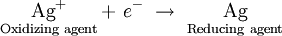# 11.20: Redox Couples

When a reducing agent donates one or more electrons, its oxidation number goes up, and the resulting species is capable of reaccepting the electrons. That is, the oxidized species is an oxidizing agent. For example, when copper metal dissolves, the copper(II) ion formed can serve as an oxidizing agent:Similarly, when an oxidizing agent such as silver ion is reduced, the silver metal can donate an electron, serving as a reducing agent:This is analogous to what we observe in the case of conjugate acids and bases. For every oxidizing agent, there corresponds some reducing agent, and for every reducing agent, there corresponds an oxidizing agent.

TABLE $$\PageIndex{1}$$: Selected Redox Couples Arranged in Order of Decreasing Strength of Oxidizing Agent.An oxidizing and reducing agent which appear on opposite sides of a half-equation constitute a redox couple. Redox couples are analogous to conjugate acid-base pairs and behave in much the same way. The stronger an oxidizing agent, the weaker the corresponding reducing agent, and the stronger a reducing agent, the weaker the corresponding oxidizing agent. Thus the strong oxidizing agent F2 produces the weak reducing agent F. Conversely, the strong reducing agent Li corresponds with the weak oxidizing agent Li+.

This type of relationship is demonstrated in Table $$\PageIndex{1}$$, where selected redox couples have been arranged in order of increasing strength of the reducing agent. As in the case of acids and bases, this table of half-equations can be used to predict which way a redox reaction will proceed. The reactions with the greatest tendency to occur are between strong oxidizing agents from the upper left and strong reducing agents from the lower right. If a line is drawn from oxidizing agent to reducing agent for such a reaction, it will have a downhill slope. Reactions with little tendency to occur involve the weak oxidizing agents at the lower left and weak reducing agents at the upper right. In such cases a line from oxidizing to reducing agent has an uphill slope. When the slope of the line is not far from horizontal, the oxidizing and reducing agents are similar in strength and their reaction will go only part way to completion.

Example $$\PageIndex{1}$$: Oxidation

Predict whether iron(III) ion, Fe3+, will oxidize copper metal. If

so, write a balanced equation for the reaction.

Solution

A line from the oxidizing agent Fe3+ to the reducing agent Cu is downhill, and so the reaction will occur. The balanced equation is the sum of the two half-equations adjusted to equalize the number of electrons transferred.

$$\ce{2Fe^{3+} + 2e^{–} -> 2Fe^{2+}}$$

$$\underline{\text{Cu} \rightarrow \text{Cu}^{2+} + \cancel{2e^–}}$$

$$\ce{2Fe^{3+} + Cu -> 2Fe^{2+} + Cu^{2+}}$$

Note that the half-equation for Cu is reversed from that in Table 1 because the reactant Cu is a reducing agent. All half-equations in Table $$\PageIndex{1}$$ have the oxidizing agent on the left.

Example $$\PageIndex{2}$$: Oxidizing Agent

Use Table 11.15 to find a reagent that will oxidize H2O (other than F2 or Cl2, which were mentioned earlier).

Solution: We must find an oxidizing agent from which a line to the reducing agent H2O has a downhill slope. The only possibilities are MnO4 or CrO72–. The latter reacts extremely slowly, but aqueous permanganate solutions decompose over a period of weeks. The concentration of MnO4 decreases because of the reaction

$$\ce{4MnO4^{–} + 12H3O^+ -> 4Mn^{2+} + 5O2 + 18H2O}$$